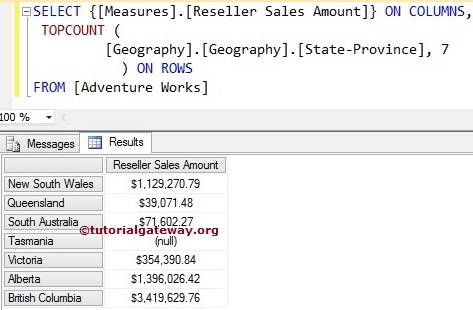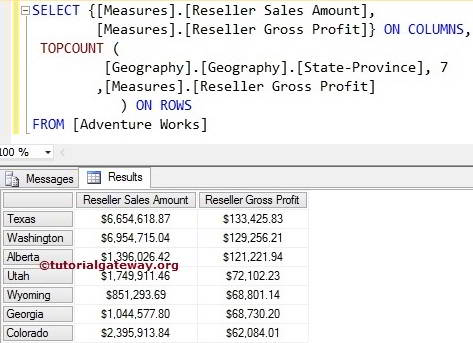# MDX TopCount Function

The Multidimensional Expression or MDX Topcount function will sort the given data in the descending order and then selects the required number of records from the sorted data.

For example, If you want to find the Top 10 performing products. Or you want to find the top 10 regions with the highest sales or to calculate the Highest Salary. We can use this MDX Topcount function with measure.

### Similarities between MDX TOPCOUNT and SQL TOP Clause

The MDX TOPCOUNT is similar to the SQL TOP Clause.

• Like Top clause, MDX Topcount extracts the required number of records in the order they initially stored.
• To extract Top 10 performing records, we have to use the Order By Clause in SQL.
• To extract Top 10 performing records, we have to add the third argument with appropriate Measure or Numeric Value.

## MDX TopCount Function Syntax

The basic syntax of the MDX Topcount function is as shown below:

`TOPCOUNT (Set_Expression, Count, Numeric_Expression)`
• Set_Expression: Any Multidimensional Expression or attributes on which you want to check.
• Count: Please provide the number of records you want to retrieve.
• Numeric_Expression: Any Multidimensional Expression or measure. It is a purely optional argument. If we used this argument, the MDX TOPCOUNT would sort the data in descending order using this Numeric_Expression. Then picks the number of records you asked for; otherwise, TOPCOUNT will select the required number of records without sorting.

In this article, we show you how to write MDX TopCount function to extract Top 7 States from the Geography table (present in the Adventure Works Cube), whose Reseller Sales amount is greater than others with examples. For this, we are going to use the below-shown data.

## MDX Topcount with Two arguments

In this example, we will show you, What happens when we miss the third argument in the Topcount function. The following query will return the first seven records from the above source in the order they appear above.

```SELECT {[Measures].[Reseller Sales Amount]} ON COLUMNS,
TOPCOUNT (
[Geography].[Geography].[State-Province]
,7
) ON ROWS

In the above MDX Query, We selected the [Reseller Sales Amount] Measure on the Columns and [State Province] column present in the geography table on Rows. Next, the Topcount function will select the Top 7 records irrespective of their measured values.No one will accept the above result as the top 7 performing states around the world.

## MDX Topcount Function Example

In this example, we will show you, What happens when we add the third argument in the MDX Topcount function. The following query will sort the records in the descending order using [Measures].[Reseller Sales Amount] and then return the first seven records from the sorted data. In short, We will get our top 7 performing states around the world.

```SELECT {[Measures].[Reseller Sales Amount]} ON COLUMNS,
TOPCOUNT (
[Geography].[Geography].[State-Province]
,7
,[Measures].[Reseller Sales Amount]
) ON ROWS

## Multiple Measures in MDX Topcount Function

What happens when we add the wrong measure as the third argument in the Topcount function?. We are going to use two measures ([Measures].[Reseller Sales Amount], [Measures].[Reseller Gross Profit]), and we assign the [Measures].[Reseller Gross Profit] as sorting argument. The following topcount query will sort the records in the descending order using [Measures].[Reseller Gross Profit] and then return the first seven records from the sorted data.

```SELECT {[Measures].[Reseller Sales Amount],
[Measures].[Reseller Gross Profit]} ON COLUMNS,
TOPCOUNT (
[Geography].[Geography].[State-Province]
,7
,[Measures].[Reseller Gross Profit]
) ON ROWS